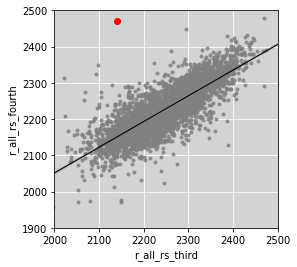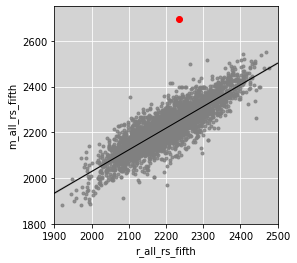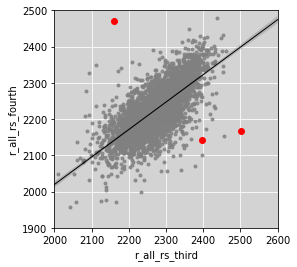# Reproducing test score graphics from The Dallas Morning News' investigation of TAKS scores#

While text-based analysis can take you far, a good graphic can help you see patterns in your data.

```import matplotlib.pyplot as plt
import pandas as pd
import seaborn as sns
import numpy as np

%matplotlib inline
```

We'll read in two years of data and combine them, roughly tracking students at the same school as they move between third and fourth grade.

From The Dallas Morning News:

Harrell Budd scored poorly in third and fifth grade. But its fourth-grade reading scores were among the best in the state

We are able to highlight Harrell Budd using its campus code of `57905115`. You have also filtered by name, etc.

```df1 = pd.read_csv("data/cfy04e4.dat", usecols=['r_all_rs', 'CAMPUS', 'CNAME'])

merged = df1.join(df2)
```
CNAME_fourth r_all_rs_fourth r_all_rs_third
CAMPUS
1902103 CAYUGA EL 2392.0 2330.0
1903101 ELKHART EL 2263.0 2285.0
1904102 FRANKSTON EL 2242.0 2299.0
```fig, ax = plt.subplots(figsize=(4,4))

ax.set_xlim(2000, 2500)
ax.set_ylim(1900, 2500)
ax.set_facecolor('lightgrey')
ax.grid(True, color='white')
ax.set_axisbelow(True)

sns.regplot('r_all_rs_third',
'r_all_rs_fourth',
data=merged,
marker='.',
line_kws={"color": "black", "linewidth": 1},
scatter_kws={"color": "grey"})

highlight = merged.loc
plt.plot(highlight.r_all_rs_third, highlight.r_all_rs_fourth, 'ro')
```
`[<matplotlib.lines.Line2D at 0x121762320>]````highlight
```
```CNAME_fourth       HARRELL BUDD EL
r_all_rs_fourth               2470
r_all_rs_third                2140
Name: 57905115, dtype: object```

This time we'll only read in one year of data - 2004 - and compare the math and reading scores at each school.From The Dallas Morning News:

Sanderson's fourth-grade math scores were exceedingly low. Its fifth-grade scores were No. 1 in the state.

We are able to highlight Sanderson using its campus code of `101912236`. You have also filtered by name, etc.

```df = pd.read_csv("data/cfy04e5.dat", usecols=['m_all_rs', 'r_all_rs', 'CAMPUS', 'CNAME'])
```
CNAME_fifth r_all_rs_fifth m_all_rs_fifth
CAMPUS
1902103 CAYUGA EL 2308.0 2317.0
1903101 ELKHART EL 2193.0 2153.0
1904102 FRANKSTON EL 2288.0 2256.0
```fig, ax = plt.subplots(figsize=(4,4))

ax.set_xlim(1900, 2500)
ax.set_ylim(1800, 2750)
ax.set_facecolor('lightgrey')
ax.grid(True, color='white')
ax.set_axisbelow(True)

sns.regplot('r_all_rs_fifth',
'm_all_rs_fifth',
data=df,
marker='.',
line_kws={"color": "black", "linewidth": 1},
scatter_kws={"color": "grey"})

highlight = df.loc
plt.plot(highlight.r_all_rs_fifth, highlight.m_all_rs_fifth, 'ro')
```
`[<matplotlib.lines.Line2D at 0x12033e6d8>]````highlight
```
```CNAME_fifth       SANDERSON EL
r_all_rs_fifth            2235
m_all_rs_fifth            2696
Name: 101912236, dtype: object```

This time we'll see how third- and fourth-graders performed at the same school in the same year. From The Dallas Morning News:

Garza's third-grade students, most of whom have problems with English, finished in the top 2 percent of the state in reading.

```df1 = pd.read_csv("data/cfy04e4.dat", usecols=['r_all_rs', 'CAMPUS', 'CNAME'])
merged = df1.join(df2)
```
CNAME_fourth r_all_rs_fourth r_all_rs_third
CAMPUS
1902103 CAYUGA EL 2392.0 2410.0
1903101 ELKHART EL 2263.0 2256.0
1904102 FRANKSTON EL 2242.0 2284.0
```fig, ax = plt.subplots(figsize=(4,4))

ax.set_xlim(2000, 2600)
ax.set_ylim(1900, 2500)
ax.set_facecolor('lightgrey')
ax.grid(True, color='white')
ax.set_axisbelow(True)

sns.regplot('r_all_rs_third',
'r_all_rs_fourth',
data=merged,
marker='.',
line_kws={"color": "black", "linewidth": 1},
scatter_kws={"color": "grey"})

highlight = merged.loc[[31901124, 57905115, 57920108]]
plt.plot(highlight.r_all_rs_third, highlight.r_all_rs_fourth, 'ro')
```
`[<matplotlib.lines.Line2D at 0x1211d7c18>]````highlight
```
CNAME_fourth r_all_rs_fourth r_all_rs_third
CAMPUS
31901124 GARZA EL 2142.0 2398.0
57905115 HARRELL BUDD EL 2470.0 2160.0
57920108 WILMER EL 2168.0 2501.0
```
```